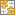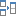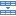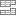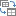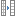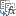# MAX IF in Excel to get highest value with conditions

The article shows a few different ways to get the max value in Excel based on one or several conditions that you specify.

In our previous tutorial, we looked at the common uses of the MAX function which is designed to return the largest number in a dataset. In some situations, however, you may need to drill down into your data further to find the max value based on certain criteria. This can be done by using a few different formulas, and this article explains all possible ways.

## Excel MAX IF formula

Until recently, Microsoft Excel did not have a built-in MAX IF function to get the maximum value based on conditions. A while ago, they introduced MAXIFS, and now the users of Excel 2019 and Excel 2016 included with Office 365 subscriptions can do conditional max an easy way.

In Excel 2013 and earlier versions, you still have to create your own array formula by combining the MAX function with an IF statement:

{=MAX(IF(criteria_range=criteria, max_range))}

To see how this generic MAX IF formula works on real data, please consider the following example. Supposing, you have a table with the long jump results of several students. The table includes the data for three rounds, and you are looking for the best result of a particular athlete, say Jacob. With the student names in A2:A10 and distances in C2:C10, the formula takes this shape:

`=MAX(IF(A2:A10="Jacob", C2:C10))`

Please keep in mind that an array formula must always be entered by pressing the Ctrl + Shift + Enter keys simultaneously. As the result, it is automatically surrounded with curly brackets like shown in the screenshot below (typing the braces manually won't work!).

I real-life worksheets, it's more convenient to input the criterion in some cell, so that you can easily change the condition without changing the formula. So, we type the desired name in F1 and get the following result:

`=MAX(IF(A2:A10=F1, C2:C10))`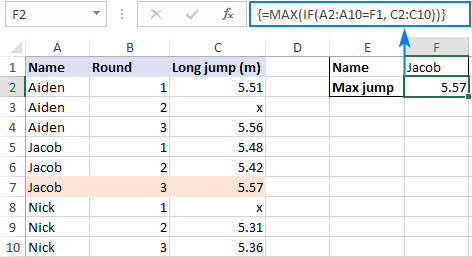### How this formula works

In the logical test of the IF function, we compare the list of names (A2:A10) with the target name (F1). The result of this operation is an array of TRUE and FALSE, where the TRUE values represent names that match the target name (Jacob):

`{FALSE;FALSE;FALSE;TRUE;TRUE;TRUE;FALSE;FALSE;FALSE}`

For the value_ if_true argument, we supply the long jump results (C2:C10), so if the logical test evaluates to TRUE, the corresponding number from column C is returned. The value_ if_false argument is omitted, meaning will just have a FALSE value where the condition is not met:

`{FALSE;FALSE;FALSE;5.48;5.42;5.57;FALSE;FALSE;FALSE}`

This array is fed to the MAX function, which returns the maximum number ignoring the FALSE values.

Tip. To see the internal arrays discussed above, select the corresponding part of the formula in your worksheet and press the F9 key. To exit the formula evaluation mode, press the Esc key.

## MAX IF formula with multiple criteria

In situation when you need to find the max value based on more than one condition, you can either:
Use nested IF statements to include additional criteria:

{=MAX(IF(criteria_range1=criteria1, IF(criteria_range2=criteria2, max_range)))}

Or handle multiple criteria by using the multiplication operation:

{=MAX(IF((criteria_range1=criteria1) * (criteria_range2=criteria2), max_range))}

Let's say you have the results of boys and girls in a single table and you wish to find the longest jump among girls in round 3. To have it done, we enter the first criterion (female) in G1, the second criterion (3) in G2, and use the following formulas to work out the max value:

`=MAX(IF(B2:B16=G1, IF(C2:C16=G2, D2:D16)))`

`=MAX(IF((B2:B16=G1)*(C2:C16=G2), D2:D16))`

Since both are array formulas, please remember to press Ctrl + Shift + Enter to complete them correctly.

As shown in the screenshot below, the formulas produce the same result, so which one to use is a matter of your personal preference. For me, the formula with the Boolean logic is easier to read and build – it allows adding as many conditions as you want without nesting additional IF functions.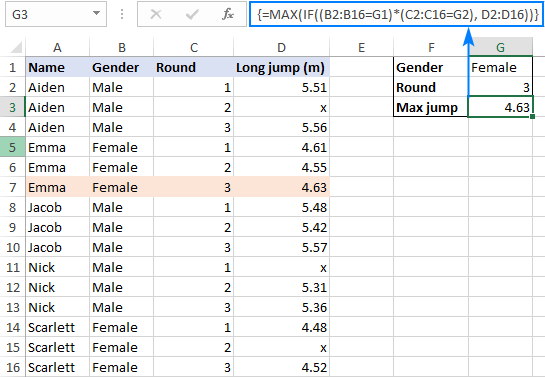### How these formulas work

The first formula uses two nested IF functions to evaluate two criteria. In the logical test of the first IF statement, we compare the values in the Gender column (B2:B16) with the criterion in G1 ("Female"). The result is an array of TRUE and FALSE values where TRUE represents data that match the criterion:

`{FALSE; FALSE; FALSE; TRUE; TRUE; TRUE; FALSE; FALSE; FALSE; FALSE; FALSE; FALSE; TRUE; TRUE; TRUE}`

In a similar fashion, the second IF function checks the values in the Round column (C2:C16) against the criterion in G2.

For the value_if_true argument in the second IF statement, we supply the long jump results (D2:D16), and this way we get the items that have TRUE in the first two arrays in corresponding positions (i.e. the items where the gender is "female" and round is 3):

`{FALSE; FALSE; FALSE; FALSE; FALSE; 4.63; FALSE; FALSE; FALSE; FALSE; FALSE; FALSE; FALSE; FALSE; 4.52}`

This final array goes to the MAX function and it returns the largest number.

The second formula evaluates the same conditions within a single logical test and the multiplication operation works like the AND operator:

When the TRUE and FALSE values are used in any arithmetic operation, they are converted into 1's and 0's, respectively. And because multiplying by 0 always gives zero, the resulting array has 1 only when all the conditions are TRUE. This array is evaluated in the logical test of the IF function, which returns the distances corresponding to the 1 (TRUE) elements.

## MAX IF without array

Many Excel users, including me, are prejudiced against array formulas and try to get rid of them wherever possible. Luckily, Microsoft Excel has a few functions that handle array natively, and we can use one of such functions, namely SUMPRODUCT, as kind of "wrapper" around MAX.

The generic MAX IF formula without array is as follows:

=SUMPRODUCT(MAX((criteria_range1=criteria1) * (criteria_range2=criteria2) * max_range))

Naturally, you can add more range/criteria pairs if needed.

To see the formula in action, we will be using the data from the previous example. The aim is to get the maximum jump of a female athlete in round 3:

`=SUMPRODUCT(MAX(((B2:B16=G1) * (C2:C16=G2) * (D2:D16))))`

This formula is competed with a normal Enter keystroke and returns the same result as the array MAX IF formula: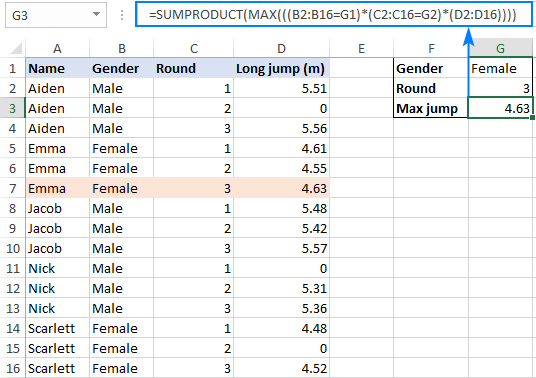Casting a closer look at the above screenshot, you can notice that invalid jumps marked with "x" in the previous examples now have 0 values in rows 3, 11 and 15, and the next section explains why.

### How this formula works

As with the MAX IF formula, we evaluate two criteria by comparing each value in the Gender (B2:B16) and Round (C2:C16) columns with the criteria in cells G1 and G2. The result are two arrays of TRUE and FALSE values. Multiplying the arrays' elements in the same positions converts TRUE and FALSE into 1 and 0, respectively, where 1 represents the items that meet both criteria. The third multiplied array contains the long jump results (D2:D16). And because multiplying by 0 gives zero, only the items that have 1 (TRUE) in the corresponding positions survive:

`{0; 0; 0; 0; 0; 4.63; 0; 0; 0; 0; 0; 0; 0; 0; 4.52}`

In case max_range contains any text value, the multiplication operation returns the #VALUE error because of which the entire formula won't work.

The MAX function takes it from here and returns the largest number that meets the specified conditions. The resulting array consisting of a single element {4.63} goes to the SUMPRODUCT function and it outputs the max number in a cell.

Note. Because of its specific logic, the formula works with the following caveats:

• The range where you search for the highest value must contain only numbers. If there are any text values, a #VALUE! error is returned.
• The formula cannot evaluate the "not equal to zero" condition in a negative data set. To find max value ignoring zeros, use either a MAX IF formula or MAXIFS function.

## Excel MAX IF formula with OR logic

To find the max value when any of the specified conditions is met, use the already familiar array MAX IF formula with the Boolean logic, but add the conditions instead of multiplying them.

{=MAX(IF((criteria_range1=criteria1) + (criteria_range2=criteria2), max_range))}

Alternatively, you can use the following non-array formula:

=SUMPRODUCT(MAX(((criteria_range1=criteria1) + (criteria_range2=criteria2)) * max_range))

As an example, let's work out the best result in rounds 2 and 3. Please pay attention that in the Excel language, the task is formulated differently: return the max value if round is either 2 or 3.

With the rounds listed in B2:B10, the results in C2:C10 and criteria in F1 and H1, the formula goes as follows:

`=MAX(IF((B2:B10=F1) + (B2:B10=H1), C2:C10))`

Enter the formula by pressing the Ctrl + Shift + Enter key combination and you will get this result: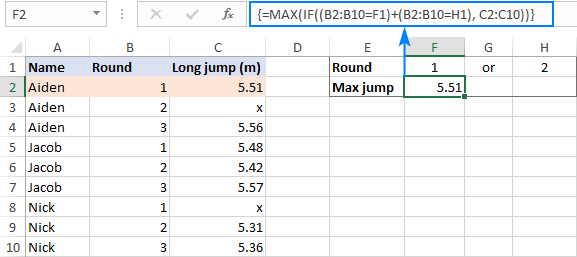The max value with the same conditions can also be found by using this non-array formula:

`=SUMPRODUCT(MAX(((B2:B10=F1) + (B2:B10=H1)) * C2:C10))`

However, we need to replace all "x" values in column C with zeros in this case because SUMPRODUCT MAX only works with numeric data: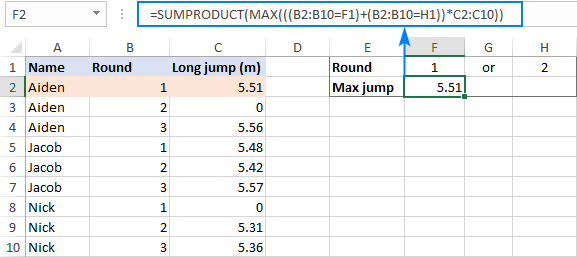### How these formulas work

The array formula works exactly the same way as MAX IF with AND logic except that you join the criteria by using the addition operation instead of multiplication. In array formulas, addition works as the OR operator:

Adding up two arrays of TRUE and FALSE (which result from checking the values in B2:B10 against the criteria in F1 and H1) produces an array of 1's and 0's where 1 represents the items for which either condition is TRUE and 0 represents the items for which both conditions are FALSE. As the result, the IF function "keeps" all the items in C2:C10 (value_if_true) for which any condition is TRUE (1); the remaining items are replaced with FALSE because the value_if_false argument is not specified.

The non-array formula works in a similar manner. The difference is that instead of IF's logical test, you multiply the elements of the 1's and 0's array by the elements of the long jump results array (C2:C10) in the corresponding positions. This nullifies the items that do not meet any condition (have 0 in the first array) and keeps the items that meet one of the conditions (have 1 in the first array).

## MAXIFS – easy way to find highest value with conditions

The users of Excel 2019 and Excel for Office 365 are free from the trouble of taming arrays to build their own MAX IF formula. These versions of Excel provide the long-awaited MAXIFS function that makes finding the largest value with conditions child's play.

In the first argument of MAXIFS, you enter the range in which the maximum value should be found (D2:D16 in our case), and in the subsequent arguments you can enter up to 126 range/criteria pairs. For example:

`=MAXIFS(D2:D16, B2:B16, G1, C2:C16, G2)`

As shown in the screenshot below, this simple formula has no problem with processing the range that contains both numeric and text values: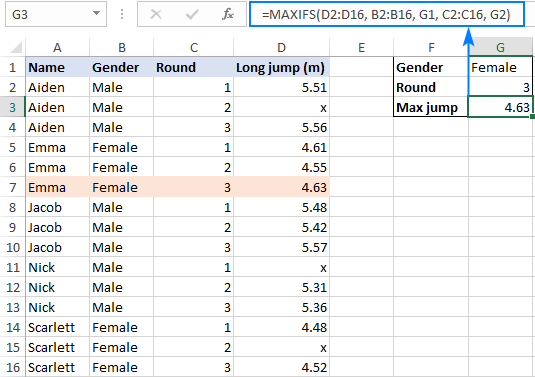That's how you can find max value with conditions in Excel. I thank you for reading and hope to see you on our blog next week!

Excel MAX IF formula examples (.xlsx file)

### 27 responses to "MAX IF in Excel to get highest value with conditions"

1.Imran Masud says:

Svetlana!!
One Caveat: Your all articles are very attractive. its consume my time eventhough i have learnt many..

•Svetlana Cheusheva (Ablebits.com Team) says:

Hi Imran,

If that's the only caveat, I think I can live with it :) And thank you for your comment, it really put a smile on my face.

2.rajesh says:

How can I print max absolute value irrespective of sign (positive or negative) and print max with sign.

3.Jackie says:

The example only showing numbers. What if we want to reverse the result? IE from your example, who has the highest jump and on what round?

•Svetlana Cheusheva (Ablebits.com Team) says:

Hi Jackie,

For this, you can use the classic INDEX MATCH formula and nest a regular MAX function in the first argument of MATCH as the lookup value.

Assuming column A contains names, column B - rounds and column C - results, the formulas would go as follows:

The person who made the highest jump:
=INDEX(\$A\$2:\$A\$10, MATCH(MAX(\$C\$2:\$C\$10), \$C\$2:\$C\$10, 0))

On what round:
=INDEX(\$B\$2:\$B\$10, MATCH(MAX(\$C\$2:\$C\$10), \$C\$2:\$C\$10, 0))

•Jackie says:

Thanks, never thought of that. Ill try

•Jenny says:

What if you want to take this concept one step further and find the person who made the highest jump, but is male. So using two sets of criteria
1. Male
2. Highest Jump
Return Value: Name

4.Tamsin Jessica Robinson-Bhaduri says:

How would you find the highest result for each person?

•Svetlana Cheusheva (Ablebits.com Team) says:

Hi Tamsin Jessica,

Simply write a MAX IF formula for the first person as explained in this tutorial, and then copy the formula down by dragging the fill handle. Be sure to use a relative reference when referring to the cell containing the person's name for the reference to adjust correctly for each row.

Alternatively, you can make a list of unique names, write a formula for the first person, and then drag the formula down to get the highest result for each person.

5.Ibrahim says:

Hi..
I have a table of data which showing list purchasing items in our stock from 2004 to date,we wanted to get the latest date of purchased for each stock, i used MAXIF function and it worked, now i want te get the lastest supplier used for each of our stock item..we have approximately 5000 items . I tried to use MAXif functions but it fails,does it work only with criterias which involve numbers only......?

6.Selva says:

Hi,
Thanks for this site which developed my basic formula skills and going on strongly.
I need to define a formula to identify the max of selected column (A1:A10) with 2 criteria, (B1:B10) shall have specific word 'B' (in D5) and (C1:C10) shall not be empty.
I've tried in 2 ways =MAXIFS(A1:A10,B1:B10,D5,C1:C10,""&"") and =MAX(IF((B1:B10=D5),(C1:C10""&""),A1:A10)) but both are showing error. Can you help me please?

•Svetlana Cheusheva (Ablebits.com Team) says:

Hi Selva,

Just use "<>" as the second criteria (non-empty):
=MAXIFS(A1:A10,B1:B10,D5,C1:C10,"<>")

•Alexander Trifuntov (Ablebits.com Team) says:

Hello Selva!
If I understand your task correctly, the following formula should work for you:

=MAXIFS(A1:A10,B1:B10,D1,C1:C10,"<>"&D2)

where D2 is empty cell.

7.Raham says:

Hi Svetlana;
1 - I encounter, MAXIF is returning a zero date (00/00/1900) if the criteria not matched.
Is it possible to get #N/A if the criteria not matched?
2 - Is it possible to combine vlookup with MAXif?

•Svetlana Cheusheva (Ablebits.com Team) says:

Hi Raham,

1. That can be done by wrapping the IF function around your MAX IF formula and using the condition for the logical test. For example:
=IF(A2:A10=F1, MAX(IF(A2:A10=F1, C2:C10)), "N/A")

2. It is possible too. For example, the below formula finds the max value in A2:A10 based on condition (B2:B10=D2) and returns a match from column C:

=VLOOKUP(MAX(IF(B2:B10=D2, A2:A10)), A2:C10, 3, FALSE)

If you need to return values from left, then use INDEX MATCH instead of VLOOKUP as shown in this thread at stackoverflow.com.

8.Rakesh Kashte says:

Hi Svetlana,
I'm very thankful for this site & excel knowledge as it has developed my excel skills.
Now i want to know, how we can determine the top 3 Max values considering this MAX function??
From this example how i would get second 'MAX JUMP' value? by formula..

9.Adly Al shanty says:

Could help me with this function I tried it many times and it doesn't work for me,I am using Exel 2013!
it always shows me the maximum value in \$F\$7:\$F\$16
Names Values "=MAX(IF(\$E\$7:\$E\$16=E7,\$F\$7:\$F\$16))"
Ahmed 1 88
Ahmed 2 88
Ahmed 66 88
Tamer 1 88
Tamer 77 88
Khalid 1 88
Khalid 2 88
Rami 1 88
Rami 2 88
Rami 88 88

•Alexander Trifuntov (Ablebits.com Team) says:

Hello!
I’m sorry but your task is not entirely clear to me. Could you please describe it in more detail?
Your data has 3 columns. Which ones do you use? What data is in E and F? What result do you get and what do you want to get?

•Gwynne Wyss says:

The team member names are in E and the the team member totals are in F. They are trying to find the highest total for each of the team members individually, but the max if formula is only returning the highest total in F, which is 88.

I am having the same problem. I have have a list of 40k totals made up of 300 team members. I need to find the highest total for each individual team member, however the max if formula is giving me just the highest overall total in column F.

Any advice on how to have it only grab the highest total for an individual team member?

•Alexander Trifuntov (Ablebits.com Team) says:

Hello!
If you have Office 2019, or if you have a Microsoft 365 subscription, use the formula

=MAXIFS(F:F,E:E,I1)

where I1 - name of the member

If you have an older version MS Office -

{=MAX(IF(E:E=I1,F:F))}

This is an array formula and it needs to be entered via Ctrl + Shift + Enter, not just Enter.

10.Yza says:

How about finding the 6 highest values in a whole table (both rows and columns)?

•Svetlana Cheusheva (Ablebits.com Team) says:

Hi Yza,

For this, you can use the LARGE function. Supposing your table is in A1:D20, enter the following formula in the topmost cell where you want the results to appear, and then drag it down through 5 more cells:

=LARGE(A1:D20, ROWS(A\$2:A2))

The ROWS function is used to dynamically generate the k argument for LARGE, which determines the k-th highest value to return.

11.Choun Sina says:

Hello Dear
why ? My office can't do maxifs formula excel.

Thanks

•Alexander Trifuntov (Ablebits.com Team) says:

Hello!
This feature is available on Windows or Mac if you have Office 2019, or if you have a Microsoft 365 subscription.

12.Conor H says:

Hi Svetlana,
This article was really, really helpful! Thank you so much for walking through how this formula can be used in various combinations in such detail.

I'm currently working on a problem and am using one of these conditional functions to solve it, but it's giving me some unexpected results and for the life of me I can't figure out why it's going wrong.

I need to work out the MAX and MIN values for various samples but I want to exclude smaller data points - this is my criteria. I have to do this for 60 samples of varying size so I want to apply a consistent threshold across them all - I've decided to exclude data points that are below half the average for each sample.

The issue seems to be, when combining with INDEX MATCH I also need to apply the same criteria to the MATCH lookup array, otherwise it could return a result of one of the excluded data points that has the same max value (which in this case is a percentage so is a strong possibility).

This is the formula I'm playing with:
=INDEX(Full_sample[Country],MATCH(MAX(IF(Full_sample[# Requested]>(AVERAGE(Full_sample[# Requested])/2),Full_sample[Country Response rate])),((Full_sample[# Requested]>(AVERAGE(Full_sample[# Requested])/2))*(Full_sample[Country Response rate])),0))

Currently this formula indexes a country whose sample size is only 1, when half the average of the full sample is 12. When I break the formula down into constituent parts the MAX formula seems to work fine so I believe the issue lies with the MATCH lookup array and adding the AVERAGE calculation within it. The syntax of telling it to only look at response rates where the # requested > half the average seems correct, but I don't think it likes having that additional formula within the lookup array?
I've tried incorporating SUMPRODUCT within the MATCH and got slightly better results, but still incorrect. And strangely, when I alter the criteria to AVERAGE*2 or write in a hard number it seems to work correctly (maybe).

I've spent hours trying to solve this but am really stuck. I would very much appreciate your advice on how to incorporate this kind of criteria into the MATCH lookup array to make sure it is only indexing the values that correspond with the MAX criteria.

Thanks

•Conor H says:

[UPDATE]

I believe I have found a solution so wanted to post it before you spent any time on my above query. However, it seems a bit convoluted so I would still appreciate your advice on whether this is the best way to approach it or if you would recommend something else.

I ended up creating another nested IF within the lookup array where the TRUE value = 1 and match that to a criteria of 1. So rather than starting with the MAX (or MIN) as my match criteria, I incorporated my MAX and MINs into my IF lookup array. So far this appears to give me the correct result.

So the formula now is:
=INDEX(Full_sample[Country],MATCH(1,IF(Full_sample[# Requested]>(AVERAGE(Full_sample[# Requested])/2),IF(Full_sample[Country Response rate]=MIN(Country_Full_sample[F HQ Country Response rate]),1)),0))

I've used a MIN example here because it presents another issue, albeit one I'm prepared to live with. It could also happen in my MAX calculations but is less common.
The above formula returns a country whose response rate was 0, but at least where it's sample size was above half the average of the population (>12), whereas previously it was returning a country whose response rate was also 0 but sample size was only 1. However, there are 3 countries above the requested threshold who have response rates of 0. Ideally, I would like the indexed result to return the country who had the largest sample above the threshold whose response rate was 0 (in this case). This would tell me which country performed more objectively worse (or better in MAX cases), as it would take into account both the absolute size of the sample and the response rate.

Perhaps adding another ">" equation or incorporating the LARGE function may work, but I suspect this may make it more convoluted than is necessary. I've already set a minimum threshold so I could just as easily say that any country with the lowest response rate is the same as another.

Ultimate Suite for Microsoft Excel